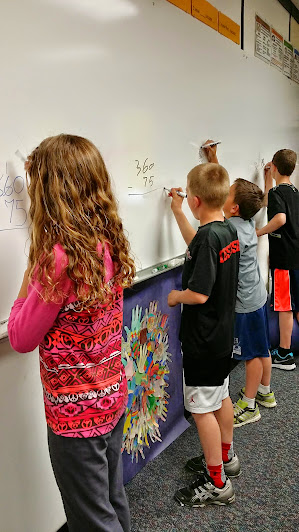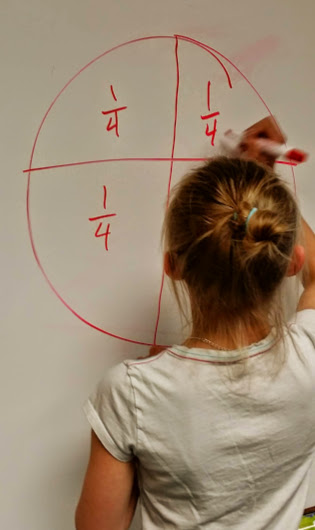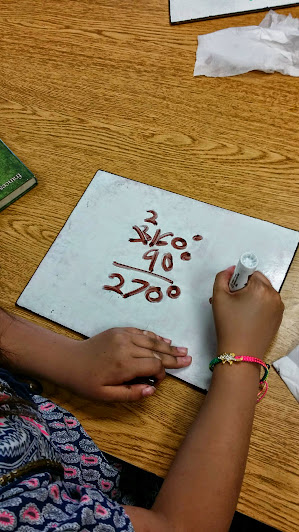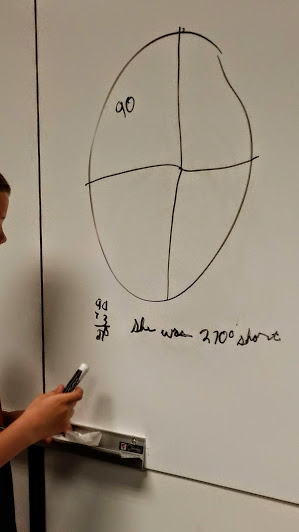# Adding up Angles

9 teachers like this lesson
Print Lesson

## Objective

SWBAT determine unknown angle degrees and solve word problems using the four operations.

#### Big Idea

In this lesson, students are up and out of their seats in an engaging problem solving strategy. Students deepen their understanding about angles as they talk with peers about various strategies to determine angle measurements.

## Number Hook

5 minutes

I begin this lesson with a number hook I call 99.  I start by instructing students to write down any two different numbers from 1 to 9. Then reverse the two numbers.  Students should have two two-digit numbers.  Subtract the smaller number from the larger one.  Take the result, reverse the digits, and add that number to the one you got when you subtracted.

The answer is 99.

For example: 72 reverses to make 27.  Subtract the smaller (27) from the larger (72): 45. Reverse these digits to make 54.  Add this to the previous number.  The answer is 99.

## Warm up

5 minutes

To get students moving and thinking I instruct all students to make an acute angle with their arms out stretched.  Next, I ask them to make an obtuse angle and a right angle.  We look at all the angles created and I ask a student volunteer to explain in words the definition of each angle.

## Concept Development

45 minutes

To begin this lesson, I assign each student a whiteboard space around the room or a personal whiteboard. You can see in the photo below students working at their assigned white board space.  I was lucky enough to have two major walls in my classroom white boarded.Students solve word problems in this lesson, but I also want to create an atmosphere and climate in which students will be able to talk about each problem.  By getting students out of their seats, using a whiteboard, I can then pause after each question and lead a brief discussion about strategies and help students make connections between strategies and concepts.

I use the problems from this resource - angle word problems and place it under the document camera.  I start by displaying the first problem and ask students to solve the problem using picture, words, or numbers. I discourage students from writing down the answer only (particularly since many students can complete number 1 mentally) and stress that we will be having a class discussion about strategies so it will be important that all students be able to see the strategies used.  I have found that when students have a written account of their work, this also helps when they talk about their strategy used because they can refer back to their work.  I help students make connections about angles being additive. I remind them that when an angle is decomposed into two angles and you know the measurement of the original angle and one of the smaller angles, you can find the measurement of the second angle.  I ask students to explain how to do this.  Some other things I point out during the discussion are non-overlapping angles, angles in real life, and inverse operations.

This shows student work from the ice skating problem and the quarter turn. Notice that all three students use a different strategy.I continue giving students problems from the angle word problem sheet until we have completed the first page together and discussed them all.  Next, I direct students to complete the second page on their own on a piece of paper.  I encourage students to think about strategies talked about and refer to those if they get stuck.

Listen in as this student tries to make sense of the information in the problem about the propeller blades. She is confused by the extra information in the problem.

When students are finished with the problems from this page, I ask them to solve the missing angles from this page-- missing angle sheet.

When all students are finished, we correct this and the problem solving page together. I ask students to share their thinking and strategies.

This is a great lesson that does not necessarily directly teach students about angles as additive, but students understand this concept through the problems and discussion in this lesson.